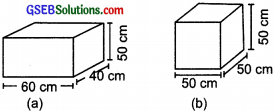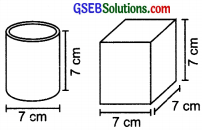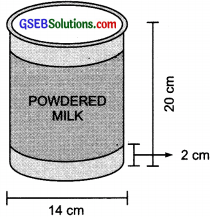# GSEB Solutions Class 8 Maths Chapter 11 Mensuration Ex 11.3

Gujarat Board GSEB Textbook Solutions Class 8 Maths Chapter 11 Mensuration Ex 11.3 Textbook Questions and Answers.

## Gujarat Board Textbook Solutions Class 8 Maths Chapter 11 Mensuration Ex 11.3

Question 1.
There are two cuboidal boxes as shown in the adjoining figure. Which box requires the lesser amount of material to make?Solution:
Total surface area of the cuboid (a)
= 2[lb + bh + hl]
= 2[(60 × 40) + (40 × 50) + (50 × 60)] cm2
= 2[2400 + 2000 + 3000] cm2
= 2[7400 cm2] = 14800 cm2

Total surface area of the cuboid (b)
= 2[lb + bh + hl]
= 2[(50 × 50) + (50 × 50) + (50 × 50)]
= 2[2500 + 2500 + 2500] cm2
= 2[7500 cm2] = 15000 cm2

Since the total surface area of the second (b) is more.
∴ Cuboid (a) will require lesser material.Question 2.
A suitcase with measures 80 cm × 48 cm × 24 cm is to be covered with a tarpaulin cloth. How many metres of tarpaulin of width 96 cm is required to cover 100 such suitcases?
Solution:
Total surface area of a suitcase
= 2 (lb + bh + hl)
= 2(80 × 48 + 48 × 24 + 24 × 80)
= 2(3840 + 1152 + 1920) cm2
= 2(6912) cm2
= 13824 cm2

∴ Total S.A. of 100 suitcases
= 100 × 13824 cm2
= 1382400 cm2
Surface area of 1 m of tarpaulin
= 100 cm × 96 cm = 9600 cm2
i.e., 1 m of tarpaulin can cover 9600 cm2 surface

∴ $$\frac{1382400}{9600}$$ metres of tarpaulin will cover the total surfaces of 100 suitcases
i.e., $$\frac{1382400}{9600}$$ or 144 metres of tarpaulin will be required to cover 100 suitcases.

Question 3.
Find the side of a cube whose surface area is 600 cm2.
Solution:
Let the side be x cm.
∴ Total S.A. of the cube = 6 × x2 cm2
But the S.A. of the cube = 600 cm2
∴ 6x2 = 600
or x2 = $$\frac{600}{6}$$ = 100
or x2 = 102 ⇒ x = 10
∴ The required side of the cube = 10 cmQuestion 4.
Rukhsar painted the outside of the cabinet of measure 1 m × 2 m × 1.5 m. How much surface area did she cover if she painted all except bottom of the cabinet?
Solution:
Total surface area = 2[lb × bh + hl]
But bottom is not painted,
i.e., Area not painted = lb
∴ Total S.A. to be painted = 2(bh + hl) + lb
= 2[(2 × 1.5) + (1.5 × 1)] + (2 × 1) m2
= 2[3 + 1.5] + 2 m2
= 2[4.5] + 2 m2
= 9 + 2 m2
= 11 m2

Question 5.
Daniel is painting the walls and ceiling of a cuboidal hall with length, breadth and height of 15 m, 10 m and 7 m respectively. From each can of paint 100 m2 of area is painted. How many cans of paint will she need to paint the room?
Solution:
Area to be painted
= [Area of 4-walls] + Area of ceiling
= 2[Perimeter × Height] + l × b
= 2[(15 + 10) × 7] + 15 × 10 m2
= 2[25 × 7] + 150 m2
= 2 × 175 + 150 m2
= 350 + 150 m2
= 500 m2
Since 1 can of paint covers 100 m2 area.
∴ Number of cans required = $$\frac{500}{100}$$ = 5Question 6.
Describe how the two figures at the right are alike and how they are different. Which box has larger surface area?Solution:
Similarity: Both have the same height.
Difference: Cylinder has curved and circular surfaces.
Cube: All faces are identical squares. Lateral surface area of the cylinder = 2πrh
= 2 × $$\frac{22}{7}$$ × $$\frac{7}{2}$$ × 7 cm2 = 7 × 22 cm2 = 154 cm2
Lateral surface area of the cube = 4l2
= 4 × (7 × 7) cm2
= 4 × 49 cm2
= 196 cm2
Obviously the cubical box has larger surface area.

Question 7.
A closed cylindrical tank of radius 7 m and height 3 m is made from a sheet of metal. How much sheet of metal is required?
Solution:
Here, r = 7 m and h = 3 m
∴ Total surface area = 2πr(r + h)
= 2 × $$\frac{22}{7}$$ × 7 × (7 + 3) m2
= 2 × $$\frac{22}{7}$$ × 7 × 10 m2
= 2 × 22 × 10 m2 = 440 m2
Thus, 440 m2 metal is required.Question 8.
The lateral surface area of a hollow cylinder is 4224 cm2. It is cut along its height and formed a rectangular sheet of width 33 cm. Find the perimeter of rectangular sheet?
Solution:
∵ Lateral surface area of a cylinder
= 4224 cm2 and let length of the rectangular sheet = l cm
∴ 33 × l = 4224
∴ l = $$\frac{4224}{33}$$ = 128 cm
Now perimeter of the rectangular sheet
= 2 [128 cm + 33 cm]
= 2 × 161 cm
= 322 cm

Question 9.
A road roller takes 750 complete revolutions to move once over to level a road. Find the area of the road if the diameter of a road roller is 84 cm and length is 1 m?
Solution:
The road roller is a cylinder.
Radius = $$\frac{84}{2}$$ cm = 42 cmLength (h) = 1 m = 100 cm
∴ Lateral surface area = 2πrh
= 2 × $$\frac{22}{7}$$ × 42 × 100 cm2
= 2 × 22 × 6 × 100 cm2 = 26400 cm2
∴ Area levelled by the roller in 1 revolution = 26400 cm2
or Area levelled in 750 revolutions
= 750 × 26400 cm2 = $$\frac{750×26400}{100×100}$$ m2
= 15 × 132 m2 = 1980 m2
Thus, the required area of the road = 1980 m2Question 10.
A company packages its milk powder in cylindrical container whose base has a diameter of 14 cm and height 20 cm. Company places a label around the surface of the container as shown in the figure. If the label is placed 2 cm from top and bottom, what is the area of the label?Solution:
The label is in the shape of a cylinder having 14
Radius = $$\frac{14}{2}$$ cm = 7 cm and Height = (2 + 2) cm less than the height of the container
or Height of the label = 20 cm – [2 + 2] cm = 16 cm
[∵ The label is placed 2 cm from top and bottom.]
∴ Area of the label = 2πrh
= 2 × $$\frac{22}{7}$$ × 7 × 16 cm2
= 2 × 22 × 16 cm2 = 704 cm2